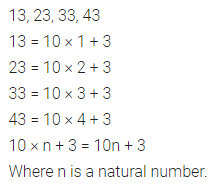ML Aggarwal Class 7 Solutions Chapter 8 Algebraic Expressions Check Your Progress for ICSE Understanding Mathematics acts as the best resource during your learning and helps you score well in your exams.

## ML Aggarwal Class 7 Solutions for ICSE Maths Chapter 8 Algebraic Expressions Check Your Progress

Question 1.
Consider the expression $$\frac { 3 }{ 2 }$$ x2y – $$\frac { 1 }{ 2 }$$ xy2 + 6x2y2.
(i) How many terms are there? What do you call such an expression?
(ii) List out the terms.
(iii) In the term $$\frac { -1 }{ 2 }$$ xy2, write down the numerical coefficient and the literal coefficient.
(iv) In the term $$\frac { -1 }{ 2 }$$ xy2, what is the coefficient of x?
Solution: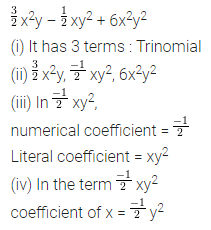Question 2.
Write the Degree of the following polynomials:
(i) $$\frac { 2 }{ 5 }$$ x3 – 7x2 – $$\frac { 1 }{ 2 }$$ x + 3
(ii) $$\frac { 2 }{ 3 }$$ xy2 – 5xy + $$\frac { 3 }{ 5 }$$ y2x2 + 2x
Solution: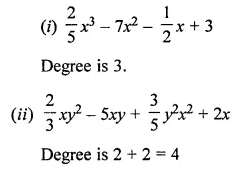Question 3.
Identify monomials, binomials and trinomials from the following algebraic expressions:
(i) 5x × y
(ii) 3 – 5x
(iii) $$\frac { 1 }{ 2 }$$ (7x – 3y + 5z)
(iv) 3x2 – 1.2xy
(v) -3x3y4z5
(vi) 5x(2x – 3y) + 7x2
Solution: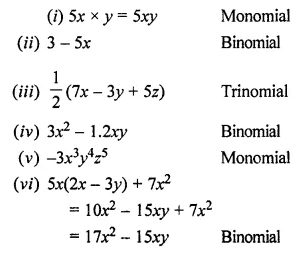Question 4.
Using horizontal method:
(i) Add x2 + y2 – 2xy, -2x2 – y2 – 2xy and 3x2 + y2 + xy
(ii) Subtract -x2 + y2 + 2xy from 2x2 – 3y2.
Solution:Question 5.
Using column method, add ab + 2bc – ca and 2ab – bc – ca and subtract 4ab + 5bc – 3ca.
Solution:Question 6.
The sides fo a triangle are 5a – 3b, 3a + 2b and 5b – 2a, find its perimeter.
Solution: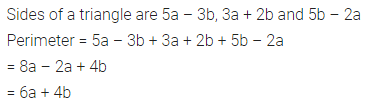Question 7.
If two adjacent sides of a rectangle are 4x +7y and 3y – x, find its perimeter.
Solution: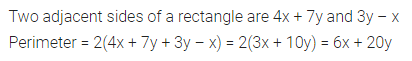Question 8.
Subtract the sum of 3x2 + 2xy – 2y2 and 5y2 – 7xy from 5x2 + 2y2 – 3xy.
Solution: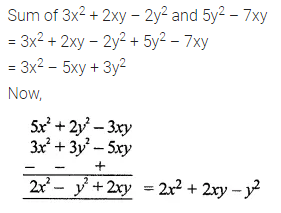Question 9.
What must be added to 5x3 – 2x2 + 3x + 7 to get 7x3 + 7x – 5?
Solution: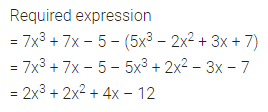Question 10.
How much is 3p – 4q + r less than 4p + 3q – 5r?
Solution:Question 11.
How much is 3a2 – 5ab + 7b2 + 3 greater than 2a2 + 2ab + 5?
Solution: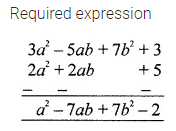Question 12.
How much should 5x3 + 3x2 – 2x + 1 be increased to get 6x2 + 7?
Solution:Question 13.
Subtract the sum of 12ab – 10b2 – 18a2 and 9ab + 12b2 + 14a2 from the sum of ab + 2b2 and 3b2 – a2.
Solution:Question 14.
when a = 3, b = 0, c = -2, find the values of:
(i) ab + 2bc + 3ca + 4abc
(ii) a3 + b3 + c3 – 3abc
Solution: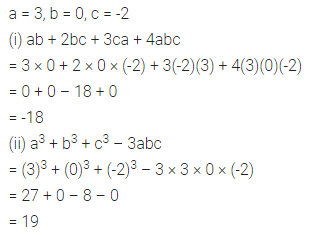Question 15.
Write the algebraic expression for the nth term of the number pattern 13, 23, 33, 43, ………..
Solution: